# Random variables: what are they and why are they needed?

This article aims at providing some intuition for what random variables are and why random variables are useful and needed in probability theory.

## Intuition for random variables

Informally speaking, random variables encode questions about the world in a numerical way.

How many heads can I get if I flip a coin 3 times?

How many people will vote the Democrats at the US presidential elections?

I want to make pizza. What is the possible overall cost of the ingredients, considering all combinations of different brands of them?

These are all examples of random variables. What a random variable does, in plain words, is to take a set of possible world configurations and group them to a number. What I mean when I say world configurations will be clearer soon, when talking about the sample space(which, appropriately, is also called universe).

I just wanted to provide a very brief informal description of random variables, but stick with me and we will dive deeper in the matter with an example!

## A simple random variable example

Suppose to flip a (balanced) two-headed coin three times. If we write down all possible outcomes, we obtain the universe (or sample space):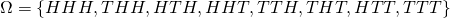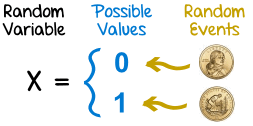In which we have identified head with H and tail with T. The first element corresponds to three heads, the following three elements correspond to two heads, the following three more correspond to one head, and the last one to no heads.

Let’s take a second to notice thatis made up of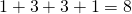items.

Now, what if I asked you how many heads you can get overall by flipping three times a coin? You would answer me by exhibiting the following set (who wouldn’t reply exhibiting a set, really!):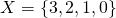Notice that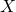is made up of only 4 elements, whereashad 8: we have reduced the amount of data to handle. (Also,was made up of more complex data, because each of its 8 elements was made up of 3 letters.)

And lo! We have stumbled upon a random variable. We had a universe of possible configurationsand, passing through a question, we have mapped them in a numerical way that’s relevant for our question. This is crucial, so I will say it once again: from, which contained a lot more information than we needed, we managed to extract the part of the data that was relevant to our study.

In a way, every time you study a phenomenon through some data, you are always using random variables to do it, because you only look at the data that’s relevant and ignore what’s not important for you at that moment. In our case, for example, we don’t care in what order the heads came, we just want two of them.

Of course, we can ask a variety of questions about the same phenomenon. In the case of the 3-coins-flipping, apart from “How many heads could we get?” we could also ask “How many tails could we get?”. It was a trivial phenomenon so there’s not much we can study about it, but try to think about a medical experimentation: there is a lot of data and several questions can be asked about it.

## Why is a random variable useful?

At this point, a random variable just seems like a very useful concept, but one could argue that reducing the amount of data is not a good enough reason to introduce a new idea.

But random variables are defined in probability theory, so they must have something to do with probabilities! Imagine we were interested in the following question “What’s the likelihood of getting 2 heads (flipping a balanced coin 3 times)?”. What is beautiful about random variables is that they work in perfect tune with the probability measure we have on!

As long as we talk about discrete cases (meaning numbers are integer: we cannot get 1.5 heads), it may look like the concept of a random variable is superfluous, because we could always go look atand see how many cases satisfy our question and how many do not. However, this is impractical for huge amounts of data, not to mention the fact that more often than not the universeis not even explicitly known. But most importantly, random variables are essential when dealing with continuous quantities and, above all, when asking more complex questions (which may involve combinations of more than one variable, for example).

## Why can’t we do away with random variables?

Mathematically speaking, a random variable is a function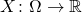Havingas output gives us a huge advantage: we can make use of all the calculus we know! We can calculate integrals, which allows us to compute the mean and variance of a phenomenon.

In a way, the abstract concept of a random variable is the price we have to pay for going beyond the “How may heads can I get by flipping 3 times a coin?”.

That’s all for now, I hope this helps in understanding the use and importance of random variables!

#### Footnotes

1. See this great math.stackexchange answer as well.